# Faults Detection and Classification under Variable Condition Using Intrinsic Time - Scale Decomposition and Neural Network

Faults Detection and Classification under Variable Condition Using Intrinsic Time - Scale Decomposition and Neural Network
Laboratoire des Matériaux et Procédés de Construction, Université de Mostagnem, Mostagnem 27000, Algeria

Laboratoire de Mécanique des Solides et des Systémes, Université de Boumerdes, Boumerdes 35000, Algeria

Corresponding Author Email:
djamaleddine.saidani@univ-mosta.dz
Page:
777-782
|
DOI:
https://doi.org/10.18280/jesa.540513
7 September 2020
|
Accepted:
25 September 2021
|
Published:
31 October 2021
| Citation

OPEN ACCESS

Abstract:

Misalignment and unbalance are a common fault occurring in the rotor system. A new approach for detecting misalignment and unbalance problems combining the intrinsic time - scale decomposition (ITD), the root mean square (RMS) and perceptron multilayer network (MLP) is proposed in this paper. Vibration signals of normal condition, misalignment horizontal, misalignment vertical and unbalance with different level are collected under different speed. ITD, nonlinear analysis of signals, was applied to decompose the vibration signals into 8 proper rotation components. The RMS values of 8 components are calculated and using as features vector. Last, the perceptron multilayer network was used for fault identification and classification. The proposed approach accurately classified and detection of unbalance and misalignment; the average accuracy achieved is 97.99%.

Keywords:

classification, intrinsic time - scale decomposition (ITD), misalignment, (RMS), unbalance

1. Introduction

Misalignment and imbalance are the most common failures of rotating machinery . According to statistics, more than 80% of rotating machine faults is due to unbalance and misalignments . Therefore, it is important to detect these faults at an early stage to minimize maintenance cost and catastrophic accidents [3, 4].

Misalignment and unbalance of rotating shafts are common cause of vibration in rotating machinery [5, 6]. Thus, the most powerful way to detect such faults is vibration monitoring and analysis .

Feature extraction is a key step in traditional fault diagnosis methods based on vibration signal , the extraction of features from vibration signals affected by the non-linearity and non-stationary [9, 10].

Recently an advanced signal processing methods using for features extraction from the vibration signals of rotating machinery . Wavelet transforms (WT) [12, 13], empirical mode decomposition (EMD) or Hilbert-Huang transform (HHT) [14, 15], local mean decomposition (LMD) , and intrinsic time-scale decomposition (ITD) .

EMD or HHT a powerful technique for non-linear and non-stationary signal processing, is superior to Fourier transform and WT and LMD in the field of machinery fault diagnosis .

The intrinsic time-scale decomposition (ITD) is superior to the EMD in reducing invalid components and mode mixing , and its calculation time is much lesser than that of the EMD method .

Different neural network classifier used the extracted features as input vectors like Multi-layer Perceptron (MLP) , k-nearest neighbor (KNN) , or support vector machine (SVM) . Comparative studies have demonstrated the efficiency of MLP over other classifier types .

In this paper, we propose a novel strategy for identify and classify the condition of the rotor system as normal, unbalanced and misaligned. Firstly ITD, nonlinear analysis of signals, are applied to decompose the vibration signals into several proper rotation components. The RMS values of these components are calculated and using as features vector. Last, the perceptron multilayer network is used for fault identification and classification.

2. Related Work

The detection and classification of unbalance and misalignment faults has been the subject of several articles in recent years as an example reference Reddy and Sekhar  developed a method using strain sensors for the detection of shaft misalignment at low speed. Wang et al.  used the torque signal for the detection of coupling misalignment in the rotor. Wang and Jiang  used laser measurements to detect misalignment. The reference  presented a study on rotor misalignment and imbalance defects. The authors observed that the unbalanced mass incentive force occurs at the first harmonic and the force associated with misalignment occurs at the second harmonic.

The signature of the defects of imbalance and misalignment is almost the same that is why difficulty of identification. For this, we will develop a new approach to identify imbalance and misalignment at different shaft speeds.

3. Methodology

The block diagram of the proposed methodology is shown in Figure 1. The signal vibration measured is devised into 20 segments equitable. Each segment is decomposed into 08 components using ITD technique. After decomposition, the root mean square of each component is calculated. The root mean squares (RMS) were used for fault detection using classifiers.

## 1.pngFigure 1. The block diagram of the proposed method

3.1 Experimental setup

As shown in Figure 2. The machinery fault simulators consist of Motor 1/4 CV DC with extended shaft, single disk rotor and two identical self-aligned ball bearing. The shaft is supported by two bearing and has a length of 520 mm with a bearing span of 390 mm. The diameter of shaft is 16 mm. VFD (Variable Frequency Drive) is used to control the speed of motor from 700 to 3600 rpm for variable speed.

## 2.png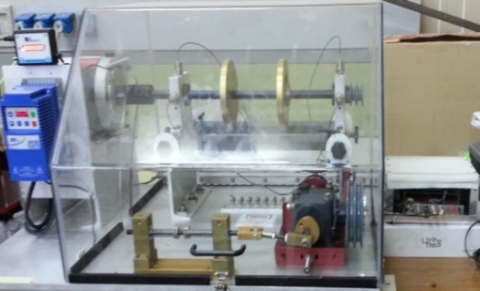Figure 2. Machinery fault simulators

3.2 Experimental results

In this work three diﬀerent types of faults are considered: Misalignment horizontal (0.5, 1 and 2mm), Misalignment vertical (0.51, 1 and 1.90mm) and unbalance (10, 15 and 20g) under three different frequency operation 15 Hz, 30 Hz and 60 Hz .

3.3 Segmentation

In this work, for augmentation data the vibration signals are divided into 20 Segments equitable. 15 segments used for training neural network and 5 segments used for testing neural network. The Table 1 below presents the number of samples vibration signals used for each class.

Table 1. The number of samples vibration signals

 Class Number of samples for Training Testing Normal 45 15 Misalignment Horizontal 135 45 Misalignment Vertical 135 45 Unbalance 135 45 Total samples 450 150

3.4 Decomposition of signal using ITD

After segmentation The Intrinsic Time-Scale Decomposition ITD method is used to decompose each segment into proper rotation components (PRCs), and a monotonic trend (we will illustrate an example in Figure 3).

The input signal Xn can be decomposed as :

Xn= Hn + Ln     (1)

where,

Xn is the input signal, Hn represents the proper rotation components (PRCs), and Ln =L Xn is the monotonic trend signal.

If the real valued signal under analysis is $\left\{\mathrm{X}_{n}, \mathrm{n} \geq 0\right\}$ and suppose $\left\{\tau_{k}, \mathrm{k}=1,2, \ldots\right\}$ be the local extreme points on $\mathrm{X}_{n}$. For convenience define, $\tau_{0},=0$ be the first sample of the signal. Let the value of the signal at $\tau_{k}$ be $\mathrm{X}\left(\tau_{k}\right)$ and value of its baseline at $\tau_{k}$ be $\mathrm{L}\left(\tau_{k}\right)$. We assume that $\mathrm{L}_{n}$ and $\mathrm{H}_{n}$ are defined on $\left[0, \tau_{k}\right]$ and $X_{n}$ is available for $\mathrm{n} \in\left[0, \tau_{k}: 2\right]$. The baseline extracting operator L is defined as a piecewise linear function on the interval $\left[\tau_{k}, \tau_{k}, 1\right]$ between the two extreme points as .

$\mathrm{L}_{n}=\mathrm{L}_{k}+\left(\frac{L_{k}+1-L_{k}}{X_{k}+1-X_{k}}\right)\left(\mathrm{X}_{n}-\mathrm{X}_{k}\right)$       (2)

where,

\begin{aligned} \mathrm{L}_{k}+1=\alpha\left(\mathrm{X}_{k}\right.&\left.+\left[\frac{\tau_{k}+1-\tau_{k}}{\tau k+2-\tau k}\right]\left(\mathrm{X}_{k}+2-\mathrm{X}_{k}\right)\right) \\ &+(1-\alpha) \mathrm{X}_{k}+1 \end{aligned}                             (3)

And $0<\alpha<1$ with a typical value of 0.5.

## 3.png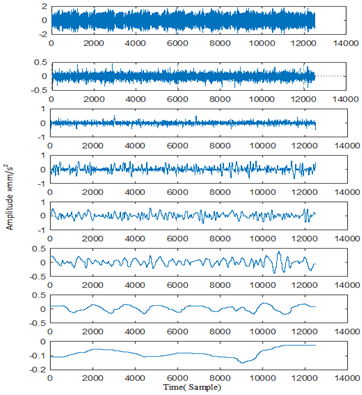Figure 3. Example illustrating ITD decomposition of vibration signal relating to normal condition a 30 Hz frequency

3.5 Feature Extraction (RMS)

After ITD decomposition, the RMS of each proper rotation component obtained by decomposition of different segments vibration signals are calculated separately. The root mean square (RMS) is defined as the square root of the mean square.

The RMS value of a discrete-time signal is the square root of the arithmetic mean of the squares of the signal sample values.

The RMS can be computed as equation following .

$\mathrm{RMS}=\sqrt{\frac{1}{\mathrm{~N}} \sum_{\mathrm{n}=1}^{\mathrm{N}} \mathrm{x}[\mathrm{n}]^{2}}$             (4)

where, n=1,2,…, N, and N is the sample size, that is, the number of observations in the sample.

## 4.pngFigure 4. Bar graph of RMS values for condition normal (a) frequency 15 Hz (b) frequency 30Hz (c) frequency 60 Hz

## 5.png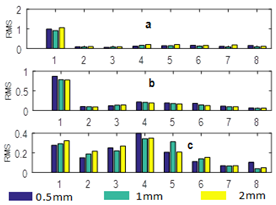Figure 5. Bar graph of RMS values for misalignment horizontal (a) frequency 15 Hz (b) frequency 30Hz (c) frequency 60 Hz

## 6.png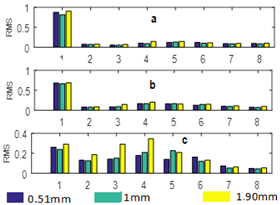Figure 6. Bar graph of RMS values for misalignment vertical (a) frequency 15 Hz (b) frequency 30Hz (c) frequency 60 Hz

## 7.png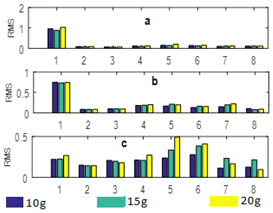Figure 7. Bar graph of RMS values for unbalance (a) frequency 15 Hz (b) frequency 30Hz (c) frequency 60 Hz

Figure 4, 5, 6 and 7 RMS values calculated of different proper rotation component of the first segment vibration signals for condition normal, misalignment horizontal, misalignment vertical and unbalance respectively.

3.6 Multilayer perceptron

In this work a multi-Layer perceptron (MLP) is used for detection and classification shaft misalignment and unbalance. It is a feedforward neural network with two layers between input and output layer. 8 input neurons and 2 output neurons. Feed forward means that data flows in one direction from input to output layer (forward). This type of network is trained with the back propagation learning algorithm.

For each neuron receiving n inputs, the neuron’s output is given by .

$\mathrm{y}_{j}=f_{k}\left(\sum_{\mathrm{i}=1}^{\mathrm{N}} w_{i j} x_{i}+b\right)$       (5)

where,

yj = neuron’s output;

fk = activation function;

wij =weight from node i to node j;

xi = neuron’s input;

=scalar.

The structure of the MLP is shown in Figure 8.

## 8.png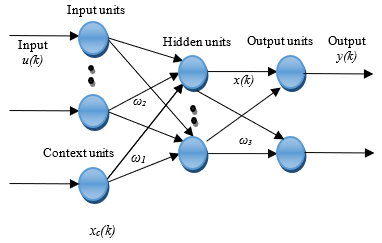Figure 8. Structure of MLP

4. Result

In this work we propose a novel approach to distinguish the different fault (misalignment horizontal (MH), misalignment vertical (MV), and unbalance) using ITD decomposition and nonlinear analysis of vibration signals. A total of 450 vibration signal segments are decomposed into 08 different components. The RMS of each component is calculated and multi-Layer perceptron (MLP) classifier is used. Our proposed approach obtained the highest classification accuracy of 97.99%, sensitivity of 94.44% and of Specificity 99.54%

The classification accuracy of the normal condition, unbalance, misalignment horizontal and misalignment vertical were 99.33%, 97.33%, 97.33% and 98.00%, respectively.

The confusion matrix of four class is presented in Table 2. 45 values were correctly classified as normal, 41 values were correctly classified as MH and 42 values were correctly classified as MV and unbalance 1 value that should have been Unbalance were classified as MV and 1 value that should have been Normal were classified as Unbalance.

Table 2. Confusion matrix

 Predicted class Accuracy (%) Sensitivity (%) Specificity (%) Normal MH MV Unbalance True class Normal 45 0 0 0 99.33 100 99.21 MH 0 41 0 0 97.33 91.11 100 MV 0 0 42 1 98.00 93.33 100 Unbalance 1 0 0 42 97.33 93.33 98.98 Average - - - - 97.99 94.44 99.54
5. Conclusions

We have proposed a new approach for misalignment and unbalance detection and classification under variable speed based on a combining the intrinsic time - scale Decomposition, RMS and perceptron multilayer. Firstly, we decompose the raw vibration signal into 8 proper rotation components using ITD, after we calculate the RMS of each component. Finally, Perceptron multilayer classifier is trained for classification and detection of misalignment, unbalance and healthy condition. Our proposed approach obtained the highest classification accuracy of 97.99%, sensitivity of 94.44% and of Specificity 99.54%.

In future, this study can be expanded to classifier other faults using other method of signal decomposition.

Nomenclature
 Subscripts MH nanoparticle MV fluid (pure water)
References

 Muszynska A. Rotordynamics [M]. New York: Tayl-or&Francis Group-CRC Press book. https://books.google.dz/books?id=TEZuBwAAQBAJ&printsec=frontcover&dq=inauthor:%22Agnieszka+Muszynska%22&hl=ar&sa=X&redir_esc=y#v=onepage&q&f=false.

 Girdhar, P., Scheffer, C. (2004). Practical Machinery Vibration Analysis and Predictive Maintenance. Elsevier, 1st Published 2004.

 Liang, L., Liu, F., Li, M., Xu, G. (2015). Manifold learning with self-organizing mapping for feature extraction of nonlinear faults in rotating machinery. Mathematical Problems in Engineering, 2015: 873905. https://doi.org/10.1155/2015/873905

 Dong, S., Chen, L., Tang, B., Xu, X., Gao, Z., Liu, J. (2015). Rotating machine fault diagnosis based on optimal morphological ﬁlter and local tangent space alignment. Shock and Vibration, 2015: 893504. https://doi.org/10.1155/2015/893504

 SpectraQuest, Inc., Applied vibration analysis training manual & laboratory exercises, Richmond: SpectraQuest, Inc. https://spectraquest.com/.

 Rao, S.S. (2011). Mechanical Vibrations. 6th Ed., Pearson – Prentice Hall, 2011.

 Sreejith, B., Verma, A.K., Srividya, A. (2008). Fault diagnosis of rolling element bearing using time-domain features and neural networks. 2008 IEEE Region 10 and the Third international Conference on Industrial and Information Systems, 2008, pp. 1-6. https://doi.org/10.1109/ICIINFS.2008.4798444

 Pan, J., Chen, J., Zi, Y., Li, Y., He, Z. (2016). Mono-component feature extraction for mechanical fault diagnosis using modified empirical wavelet transform via data-driven adaptive Fourier spectrum segment. Mechanical Systems and Signal Processing, 72-73: 160-183. https://doi.org/10.1016/j.ymssp.2015.10.017

 Abd-el-Malek, M.B., Hanna, S.S. (2018). Using ﬁlter bank property to simplify the calculations of empirical mode decomposition. Communication Nonlinear Science Numerical Simulation, 62: 429-444. https://doi.org/10.1016/j.cnsns.2018.02.035

 Moore, K.J., Kurt, M., Eriten, M., McFarland, M.D., Bergman, L.A., Vakakis, A.F. (2018). Wavelet-bounded empirical mode decomposition for measured time series analysis. Mechanical Systems and Signal Processing, 99: 14-29. https://doi.org/10.1016/j.ymssp.2017.06.005

 Li, W., Zhu, Z., Jiang, F., Zhou, G., Chen, G. (2015). Fault diagnosis of rotating machinery with a novel statistical feature extraction and evaluation method. Mechanical Systems and Signal Processing, 50-51: 414-426. https://doi.org/10.1016/j.ymssp.2014.05.034

 Al-Badour, F., Sunar, M., Cheded, L. (2011). Vibration analysis of rotating machinery using time-frequency analysis and wavelet techniques. Mechanical Systems and Signal Processing, 25(6): 2083-2101. https://doi.org/10.1016/j.ymssp.2011.01.017

 Ma, H., Yu, T., Han, Q., Zhang, Y., Wen, B., Chen, X. (2009). Time-frequency features of two types of coupled rub-impact faults in rotor systems. Journal of Sound and Vibration, 321(3-5): 1109-1128. https://doi.org/10.1016/j.jsv.2008.09.054

 Wu, T.Y., Chung, Y.L. (2009). Misalignment diagnosis of rotating machinery through vibration analysis via the hybrid EEMD and EMD approach. Smart Materials and Structures, 18(9): 1-13. https://doi.org/10.1088/0964-1726/18/9/095004

 Lin, L., Chu, F. (2012). HHT-based AE characteristics of natural fatigue cracks in rotating shafts. Mechanical Systems and Signal Processing, 26: 181-189. https://doi.org/10.1016/j.ymssp.2011.07.017

 Cheng, J., Yang, Y., Yang, Y. (20212). A rotating machinery fault diagnosis method based on local mean decomposition. Digital Signal Processing, 22(2): 356-366. https://doi.org/10.1016/j.dsp.2011.09.008

 Frei, M.G., Osorio, I. (2007). Intrinsic time-scale decomposition: time–frequency–energy analysis and real-time filtering of non-stationary signals. In: Proceedings of the Royal Society of London A: Mathematical, Physical and Engineering Sciences. The Royal Society, 321-342. https://doi.org/10.1098/rspa.2006.1761

 Peng, Z.K., Tse, P.W., Chu, F.L. (2005). A comparison study of improved Hilbert-Huang transform and wavelet transform: Application to fault diagnosis for rolling bearing. Mechanical Systems and Signal Processing, 19(5): 974-988. https://doi.org/10.1016/j.ymssp.2004.01.006

 Yuan, Z., Peng, T.T., An, D.,Cristea, D., Pop, M.A. (2019). Rolling bearing fault diagnosis based on adaptive smooth ITD and MF-DFA method. Journal of Low Frequency Noise, Vibration and Active Control, 39(4): 278-290. https://doi.org/10.1177/1461348419867012

 Hajnayeb, A., Ghasemloonia, A., Khadem, S.E., Moradi, M.H. (2011). Application and comparison of an ANN-based feature selection method and the genetic algorithm in gearbox fault diagnosis. Expert Systems with. Application, 38: 10205-10209. https://doi.org/10.1016/j.eswa.2011.02.065

 Pandya, D.H., Upadhyay, S.H., Harsha, S.P. (2013). Fault diagnosis of rolling element bearing with intrinsic mode function of acoustic emission data using APF-KNN. Expert Systems with. Application, 40: 4137-4145. https://doi.org/10.1016/j.eswa.2013.01.033

 Santos, P., Villa, L.F., Reñones, A., Bustillo, A., Maudes, J. (2015). An SVM-based solution for fault detection in wind turbines. Sensors 2015, 15: 5627-5648. https://doi.org/10.3390/s150305627

 Al-raheem, K.F., Abdul-karem, W. (2010). Rolling bearing fault diagnostics using artificial neural networks based on LaPlace wavelet analysis. International journal of Engineering, Science and Technology, 2: 278-290. https://doi.org/10.4314/ijest.v2i6.63730

 Hili, M.A., Fakhfakh, T., Haddar, M. (2006). Failure analysis of a misaligned and unbalanced flexible rotor. Journal of Failure Analysis and Prevention, 6: 73-82. https://doi.org/10.1361/154770206X117540

 Gama, A.L., Lima, W.B., Veneza, J.P.S. (2017). Detection of shaft misalignment using piezoelectric strain sensors. Exp Tech., 41: 87-93. https://doi.org/10.1007/s40799-016-0158-x

 Reddy, M.C.S., Sekhar, A.S. (2015). Detection and monitoring of coupling misalignment in rotors using torque measurements. Measurement 2015, 61: 111-122. https://doi.org/10.1016/j.measurement.2014.10.031

 Simm, A., Wang, Q., Huang, S., Zhao, W. (2016). Laser based measurement for the monitoring of shaft misalignment. Measurement, 87: 104-116. https://doi.org/10.1016/j.measurement.2016.02.034

 Wang, N., Jiang, D. (2018). Vibration response characteristics of a dual-rotor with unbalance-misalignment coupling faults: Theoretical analysis and experimental study. Mech Mach Theory, 125: 207-219. https://doi.org/10.1016/j.mechmachtheory.2018.03.009

 Signals, Multimedia, and Telecommunications Laboratory Machinery fault database 2019. http://www02.smt.ufrj.br/~offshore/mfs/page_01.html.